# How to Calculate and Solve for Apparent Porosity | Refractories

The apparent porosity is illustrated by the image below.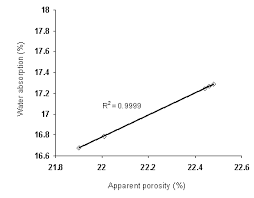To compute for apparent porosity, three essential parameters are needed and these parameters are Soaked Weight (W), Dried Weight (D) and Suspended Weight (S).

The formula for calulating apparent porosity:

AP = 100((W – D)/(W – S))

Where:

AP = Apparent Porosity
W = Soaked Weight
D = Dried Weight
S = Suspended Weight

Let’s solve an example;
Find the apparent porosity when the soaked weight is 14, the dried weight is 10 and the suspended weight is 12.

This implies that;

W = Soaked Weight = 14
D = Dried Weight = 10
S = Suspended Weight = 12

AP = 100((W – D)/(W – S))
AP = 100((14 – 10)/(14 – 12))
AP = 100(4/2)
AP = 100(2)
AP = 200

Therefore, the apparent porosity is 200%.

Nickzom Calculator – The Calculator Encyclopedia is capable of calculating the apparent porosity.

To get the answer and workings of the apparent porosity using the Nickzom Calculator – The Calculator Encyclopedia. First, you need to obtain the app.

You can get this app via any of these means:

You can also try the demo version via https://www.nickzom.org/calculator

Apple (Paid) – https://itunes.apple.com/us/app/nickzom-calculator/id1331162702?mt=8
Once, you have obtained the calculator encyclopedia app, proceed to the Calculator Map, then click on Materials and Metallurgical under Engineering.Now, Click on Refractories under Materials and Metallurgical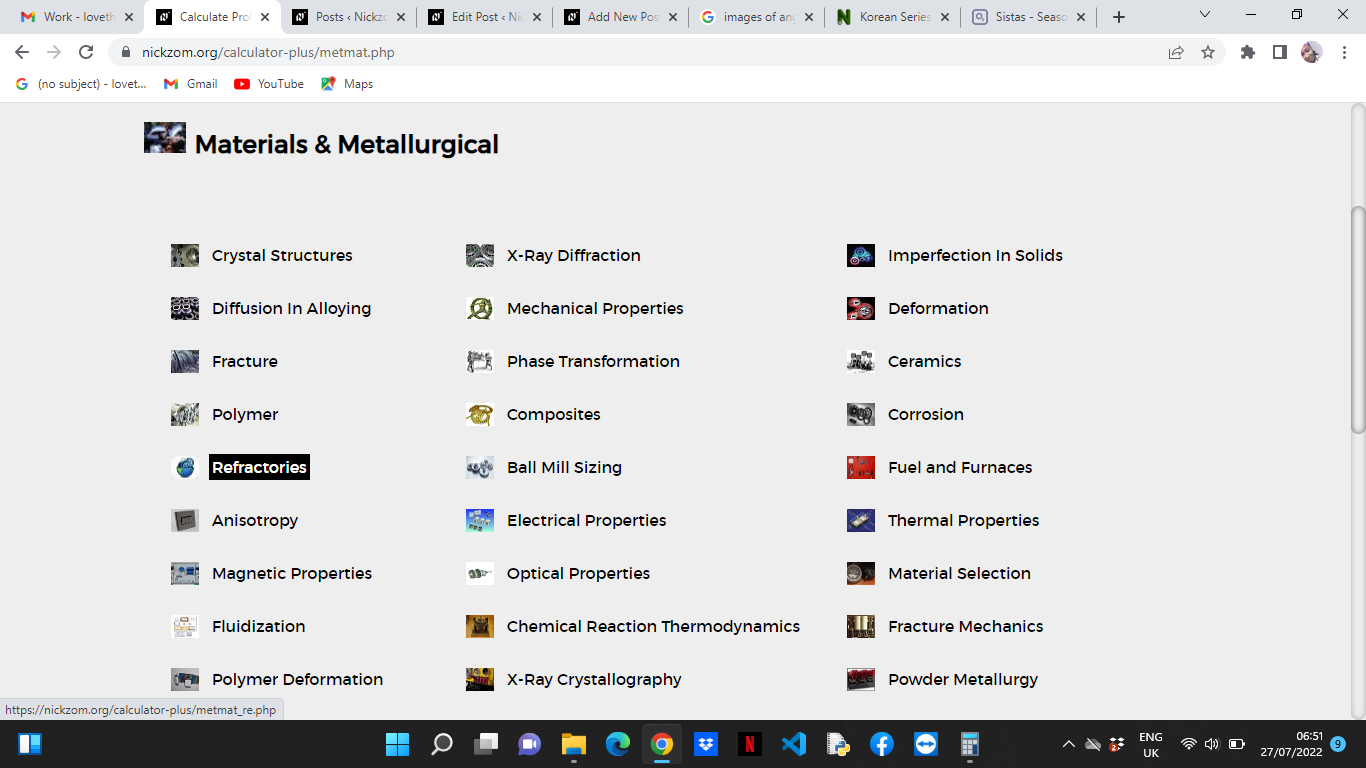Now, Click on Apparent Porosity under Refractories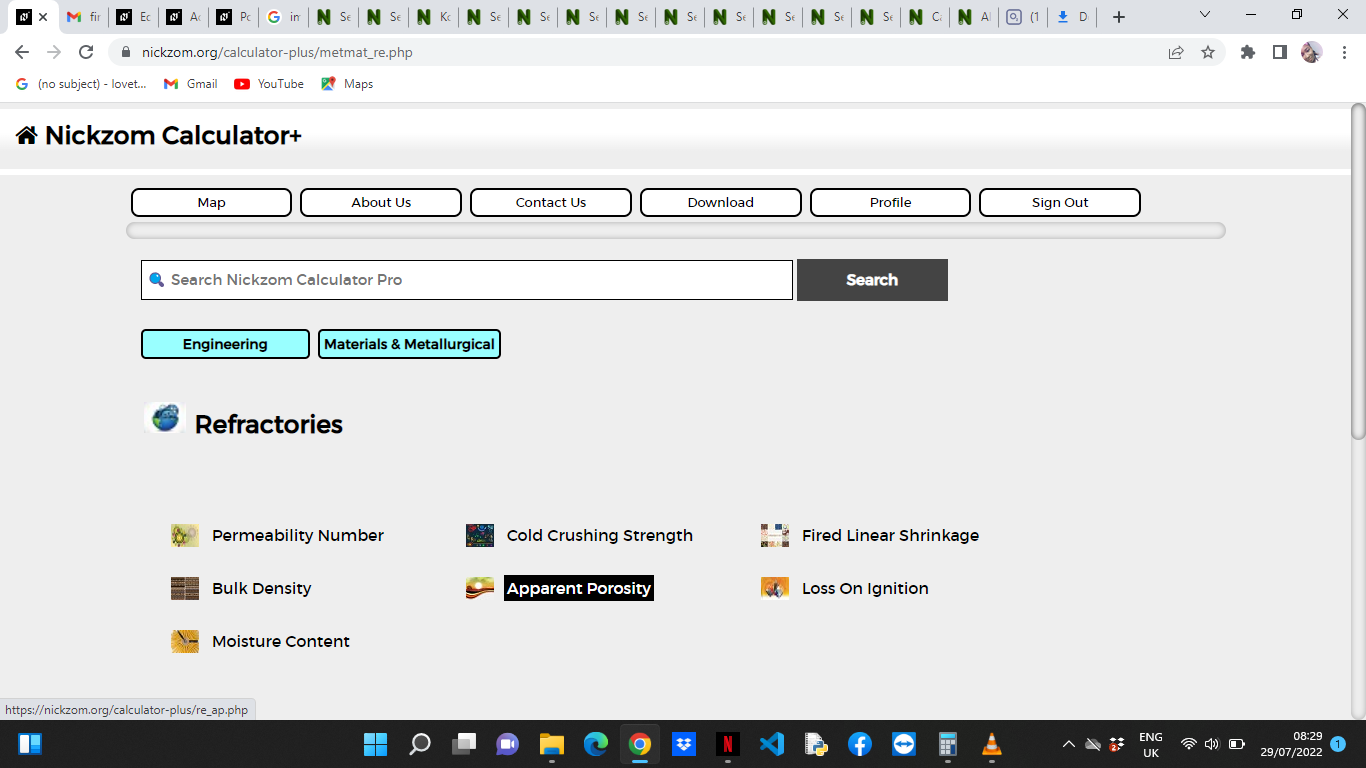The screenshot below displays the page or activity to enter your values, to get the answer for the apparent porosity according to the respective parameter which is the Soaked Weight (W), Dried Weight (D) and Suspended Weight (S).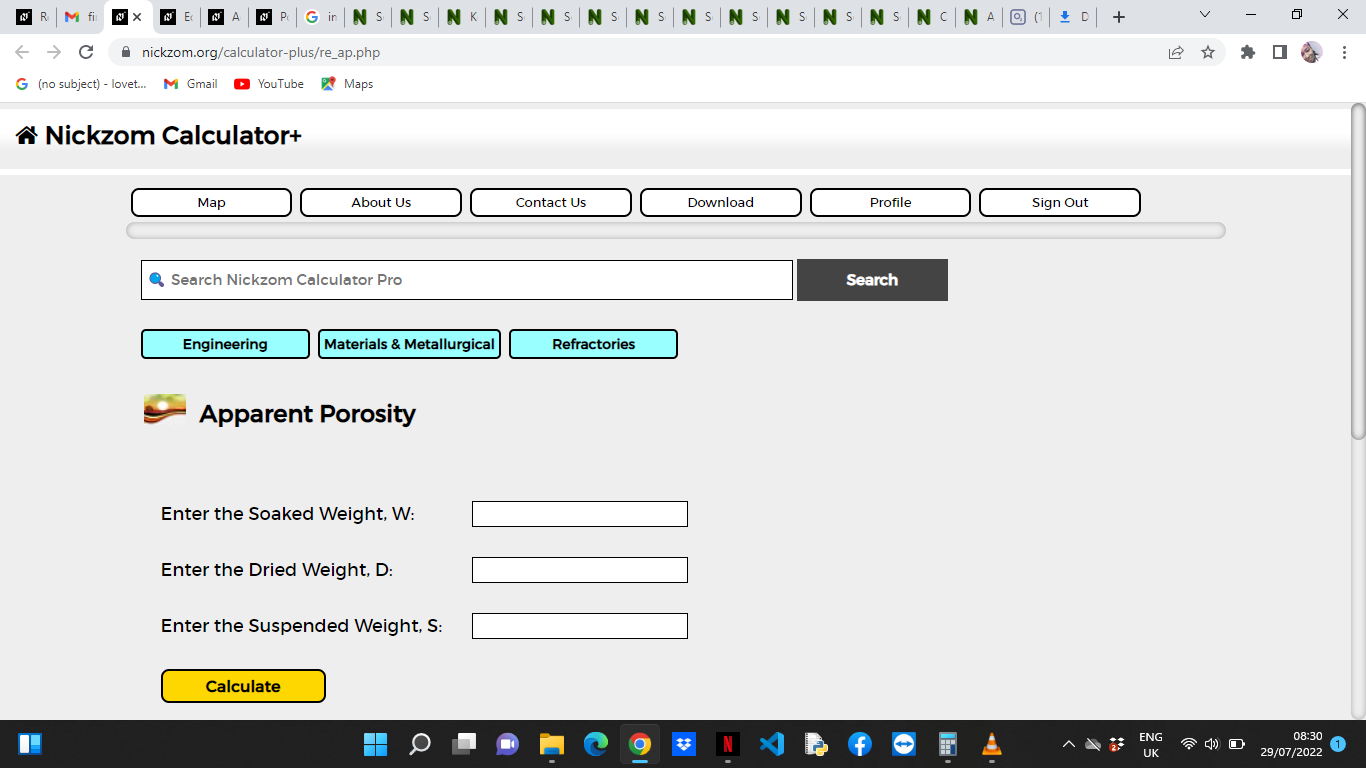Now, enter the values appropriately and accordingly for the parameters as required by the Soaked Weight (W) is 14, Dried Weight (D) is 10 and Suspended Weight (S) is 12.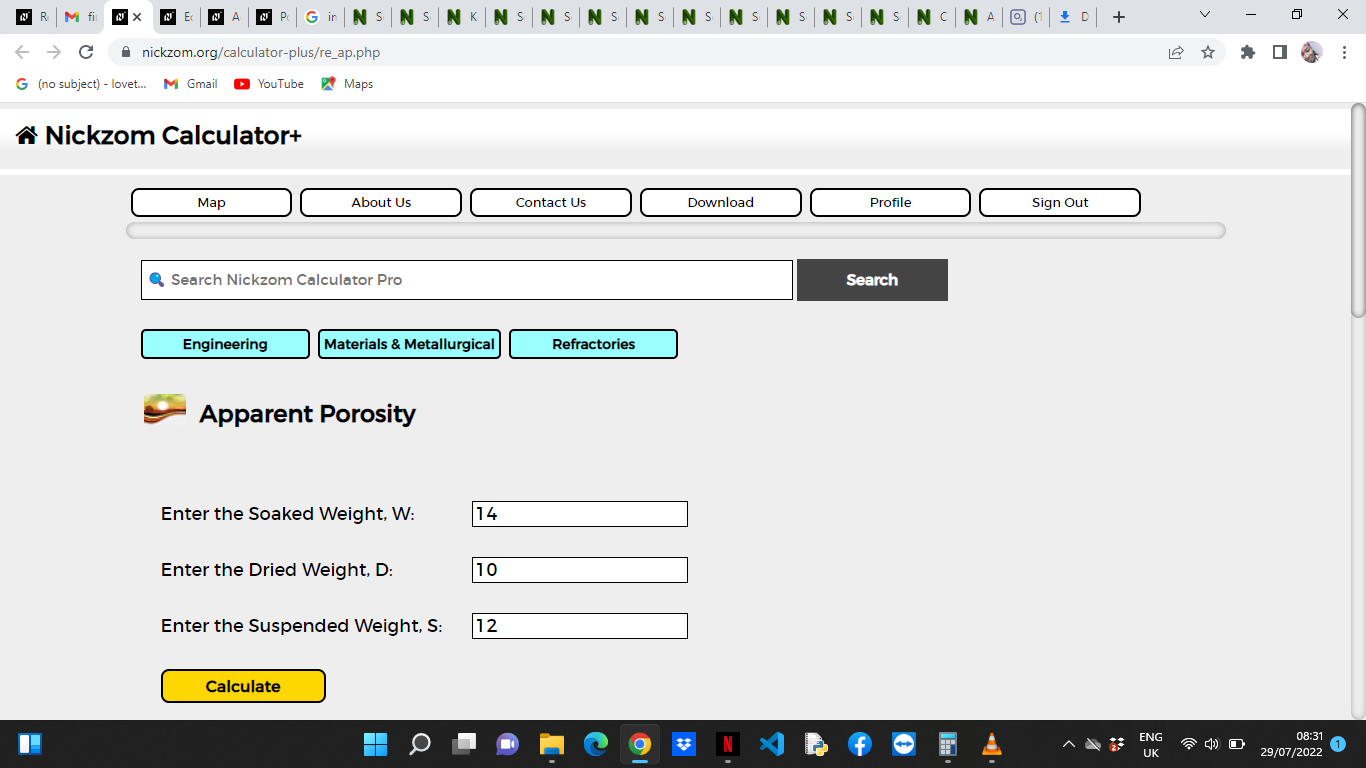Finally, Click on Calculate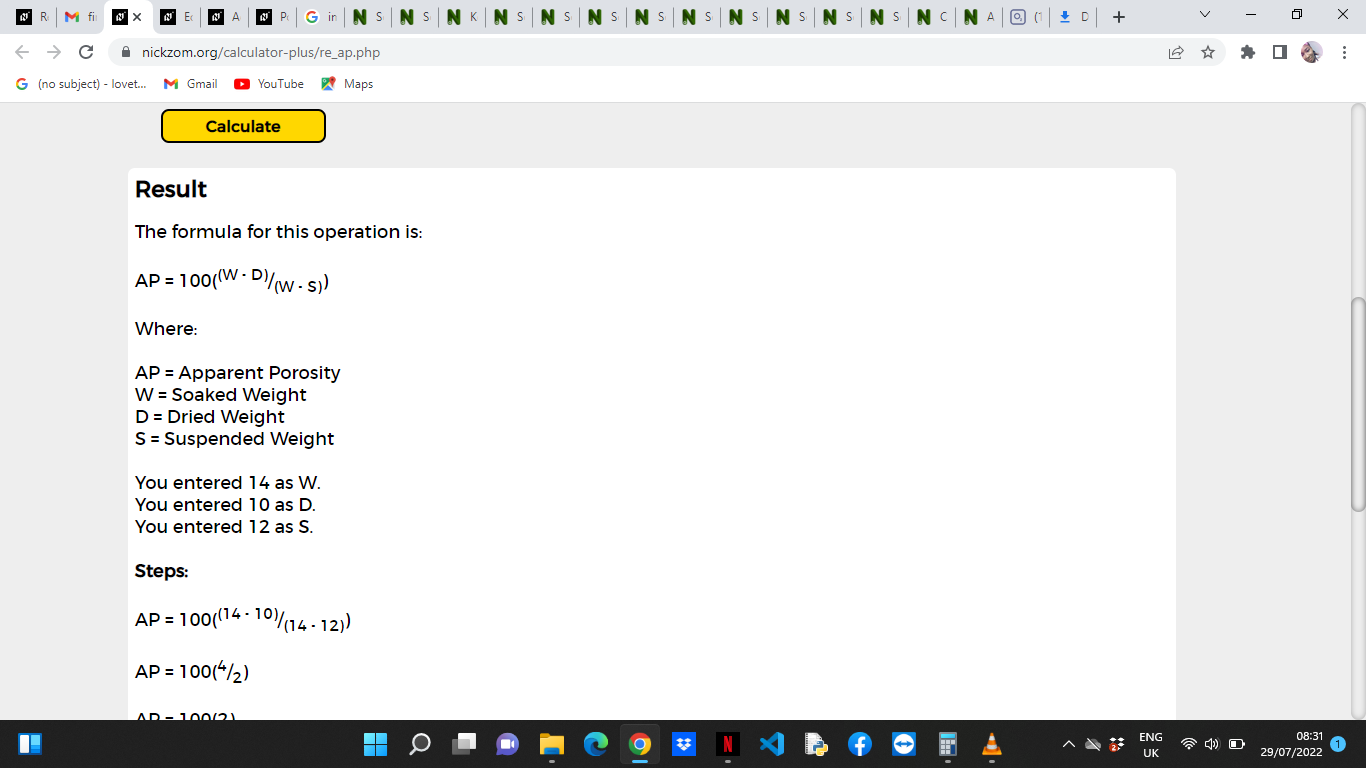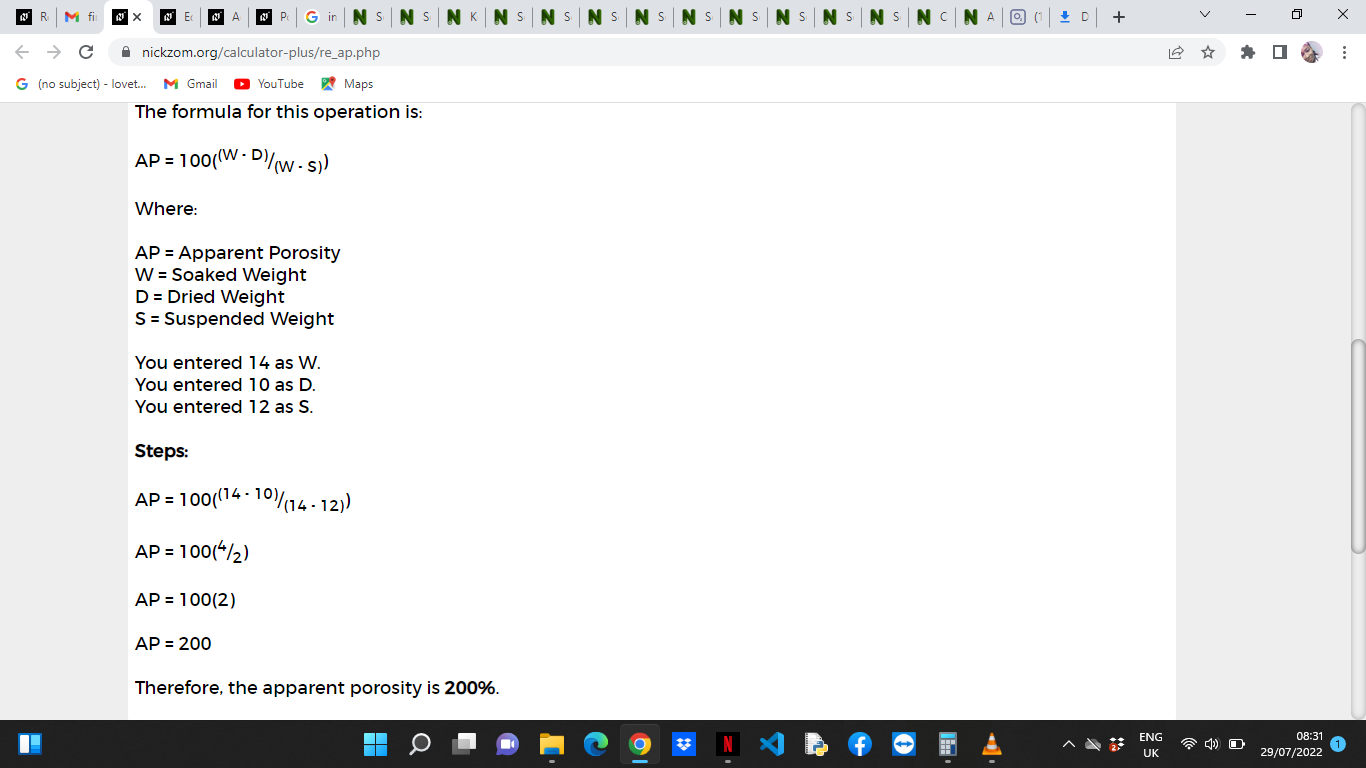As you can see from the screenshot above, Nickzom Calculator– The Calculator Encyclopedia solves for the apparent porosity and presents the formula, workings and steps too.